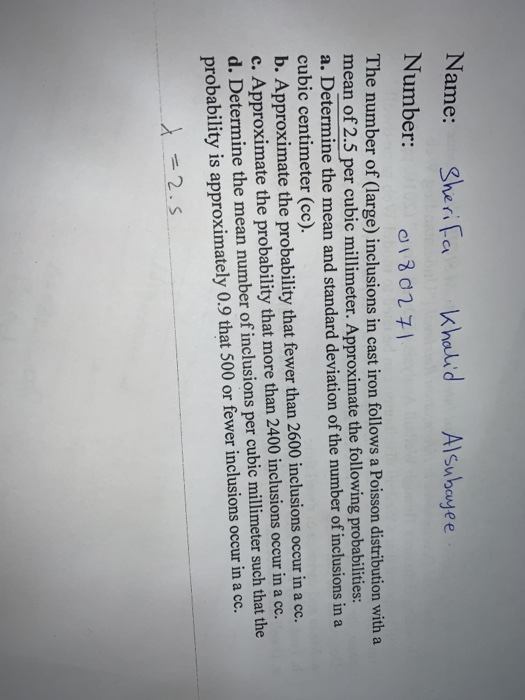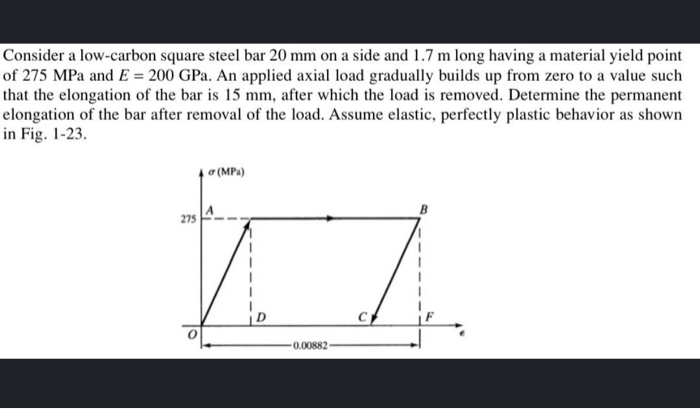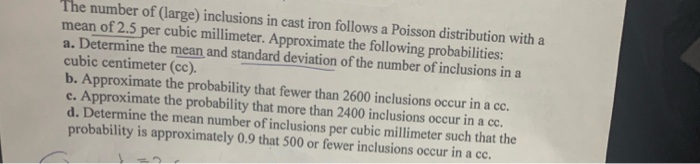# Question Solved1 AnswerSherifa Khalid Alsubayee Name: Number: on 0180271 The number of (large) inclusions in cast iron follows a Poisson distribution with a mean of 2.5 per cubic millimeter. Approximate the following probabilities: a. Determine the mean and standard deviation of the number of inclusions in a cubic centimeter (cc). b. Approximate the probability that fewer than Sherifa Khalid Alsubayee Name: Number: on 0180271 The number of (large) inclusions in cast iron follows a Poisson distribution with a mean of 2.5 per cubic millimeter. Approximate the following probabilities: a. Determine the mean and standard deviation of the number of inclusions in a cubic centimeter (cc). b. Approximate the probability that fewer than 2600 inclusions occur in a cc. c. Approximate the probability that more than 2400 inclusions occur in a cc. d. Determine the mean number of inclusions per cubic millimeter such that the probability is approximately 0.9 that 500 or fewer inclusions occur in a cc. = 2.5 Consider a low-carbon square steel bar 20 mm on a side and 1.7 m long having a material yield point of 275 MPa and E = 200 GPa. An applied axial load gradually builds up from zero to a value such that the elongation of the bar is 15 mm, after which the load is removed. Determine the permanent elongation of the bar after removal of the load. Assume elastic, perfectly plastic behavior as shown in Fig. 1-23. 4 (MPa) -0.00882 The number of (large) inclusions in cast iron follows a Poisson distribution with a mean of 2.5 per cubic millimeter. Approximate the following probabilities: a. Determine the mean and standard deviation of the number of inclusions in a cubic centimeter (cc). b. Approximate the probability that fewer than 2600 inclusions occur in a cc. c. Approximate the probability that more than 2400 inclusions occur in a ce. d. Determine the mean number of inclusions per cubic millimeter such that the probability is approximately 0.9 that 500 or fewer inclusions occur in a cc.HBOGEH The Asker · Probability and StatisticsTranscribed Image Text: Sherifa Khalid Alsubayee Name: Number: on 0180271 The number of (large) inclusions in cast iron follows a Poisson distribution with a mean of 2.5 per cubic millimeter. Approximate the following probabilities: a. Determine the mean and standard deviation of the number of inclusions in a cubic centimeter (cc). b. Approximate the probability that fewer than 2600 inclusions occur in a cc. c. Approximate the probability that more than 2400 inclusions occur in a cc. d. Determine the mean number of inclusions per cubic millimeter such that the probability is approximately 0.9 that 500 or fewer inclusions occur in a cc. = 2.5 Consider a low-carbon square steel bar 20 mm on a side and 1.7 m long having a material yield point of 275 MPa and E = 200 GPa. An applied axial load gradually builds up from zero to a value such that the elongation of the bar is 15 mm, after which the load is removed. Determine the permanent elongation of the bar after removal of the load. Assume elastic, perfectly plastic behavior as shown in Fig. 1-23. 4 (MPa) -0.00882 The number of (large) inclusions in cast iron follows a Poisson distribution with a mean of 2.5 per cubic millimeter. Approximate the following probabilities: a. Determine the mean and standard deviation of the number of inclusions in a cubic centimeter (cc). b. Approximate the probability that fewer than 2600 inclusions occur in a cc. c. Approximate the probability that more than 2400 inclusions occur in a ce. d. Determine the mean number of inclusions per cubic millimeter such that the probability is approximately 0.9 that 500 or fewer inclusions occur in a cc.
More
Transcribed Image Text: Sherifa Khalid Alsubayee Name: Number: on 0180271 The number of (large) inclusions in cast iron follows a Poisson distribution with a mean of 2.5 per cubic millimeter. Approximate the following probabilities: a. Determine the mean and standard deviation of the number of inclusions in a cubic centimeter (cc). b. Approximate the probability that fewer than 2600 inclusions occur in a cc. c. Approximate the probability that more than 2400 inclusions occur in a cc. d. Determine the mean number of inclusions per cubic millimeter such that the probability is approximately 0.9 that 500 or fewer inclusions occur in a cc. = 2.5 Consider a low-carbon square steel bar 20 mm on a side and 1.7 m long having a material yield point of 275 MPa and E = 200 GPa. An applied axial load gradually builds up from zero to a value such that the elongation of the bar is 15 mm, after which the load is removed. Determine the permanent elongation of the bar after removal of the load. Assume elastic, perfectly plastic behavior as shown in Fig. 1-23. 4 (MPa) -0.00882 The number of (large) inclusions in cast iron follows a Poisson distribution with a mean of 2.5 per cubic millimeter. Approximate the following probabilities: a. Determine the mean and standard deviation of the number of inclusions in a cubic centimeter (cc). b. Approximate the probability that fewer than 2600 inclusions occur in a cc. c. Approximate the probability that more than 2400 inclusions occur in a ce. d. Determine the mean number of inclusions per cubic millimeter such that the probability is approximately 0.9 that 500 or fewer inclusions occur in a cc.